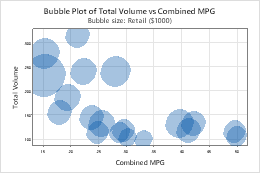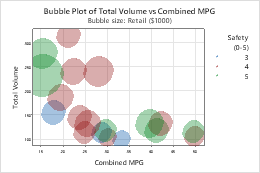# Select a bubble plot

Choose Graph > Bubble Plot, then select a bubble plot based on how you want the plot to look.

## Simple

Create a bubble plot that displays the data values for a pair of variables at their (x, y) coordinates using symbols that vary in size according to a third variable. For example, the following bubble plot shows the relationship between Total Volume (Y), Combined MPG (fuel efficiency) (X), and Retail cost (bubble size) for automobiles.